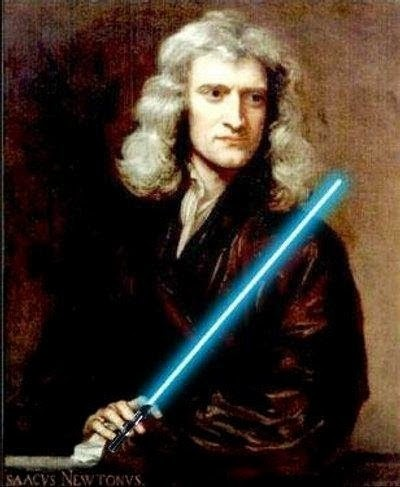# Isaac Newton,Jedi Knight

15 June 2013

When last I mentioned Newton, I noted that Sir Isaac’s genius wasn’t simply due to his laws of motion and gravity, which weren’t all that original, but rather his application and interpretation of those rules.

One of the true innovations of Newton was the application of calculus to physical problems. Newton, along with Gottfried Leibniz, is credited with laying the foundation of calculus. Leibniz focused on mathematical formalism with precise notation, and as a result the notational form we use today in calculus follows that of Leibniz. Newton on the other hand used a rather awkward geometric formalism more accessible to astronomers of his day, and he applied it to physical problems. If you’ve ever taken a calculus physics course you’ve used Newton’s approach and Leibniz’s notation.

Through calculus, Newton was able to apply his rules to general problems, which other physicists and astronomers could not. For example, it was known that circular orbits could be caused by an inverse-square force, but Newton was able to show that any conic section (circles, ellipses, parabolas and hyperbolas) could be caused by such a force. So Newton’s gravity not only accounted for the circular and elliptical orbits of planets, but also the parabolic and hyperbolic motion of comets.Wise, Newton was.

Newton’s mathematical prowess also allowed him to demonstrate approximate solutions as well. One of the aspects of his law of gravity is that its strength follows an inverse square relation when between two point masses. Of course astronomical bodies are not just simple points. They have volume and density, and aren’t even perfect spheres. Newton was not only able to show that distant spherical bodies approximated as point masses, but also how non-spherical bodies have have a gravity that is not quite inverse-square.

In particular, Newton was able to use this approach to resolve the disagreement between Kepler’s laws and the orbit of the Moon. He demonstrated that the orbital motion of the moon was due to not an inverse square (distance to the power of -2), but rather to the power of -2.016. The small deviation being due to the influence of the Sun. Thus Newton could derive both the exact and approximate answers.

There is, however, another aspect of Newton’s work that is less talked about, largely because it is so deeply ingrained in our modern scientific approach. Newton’s work was not without controversy, and much of it centered on his law of gravity. Newton proposed a gravitational attraction between any and all masses, and that the attraction obeyed an inverse-square relation. This meant that the Sun and planets moved each other, but how? Rene Descartes, for example described fluid vortices to move the planets around the Sun, but Newton simply left gravity as a nebulous kind of “action at a distance”.

Not only did Newton fail to provide a mechanism for universal gravity, he considered it a non issue. In the Principia he wrote

I have not as yet been able to deduce from phenomena the reason for these properties of gravity, and I do not feign hypotheses. For whatever is not deduced from the phenomena must be called a hypothesis; and hypotheses, whether metaphysical or physical, or based on occult qualities, or mechanical, have no place in experimental philosophy.

In other words, philosophical (physical) models are only useful if they can be tested. That probably sounds reasonable to you. So reasonable you might think it common sense. But Newton’s peers didn’t find it reasonable. For many of them, truth was contained in the philosophy of nature. For Newton, the testable answers were what mattered.

If you’ve read any philosophy, you are likely familiar with Occam’s razor. Basically, Occam’s razor states that between two philosophical arguments, the simpler one is more likely to be true. Newton’s approach can be seen as a more stringent philosophical razor. It is sometimes referred to as Newton’s Flaming Laser Sword: what cannot be settled by experiment is not worth debating. In other words, if you can’t test a hypothesis, what’s the point?

Although the term Newton’s Flaming Laser Sword wasn’t coined until this century, Newton’s approach of focusing on testable hypotheses was very influential in scientific circles. Our modern scientific methods are rooted on this idea, so much so that any approach that doesn’t rely on testable models is considered unscientific.

Another name for a flaming laser sword is a light saber. So you could say that Newton was the first scientific Jedi Knight.